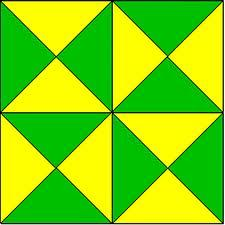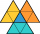# Triangle ABC

Calculate the sides of triangle ABC with area 1404 cm2 and if a: b: c = 12:7:18

a =  87.69 cm
b =  51.15 cm
c =  131.53 cm

### Step-by-step explanation:

Try calculation via our triangle calculator.Did you find an error or inaccuracy? Feel free to write us. Thank you!Tips to related online calculators
Do you have a linear equation or system of equations and looking for its solution? Or do you have a quadratic equation?
Do you want to convert length units?

#### You need to know the following knowledge to solve this word math problem:

We encourage you to watch this tutorial video on this math problem:

## Related math problems and questions:

• Circles 2Calculate the area bounded by the circumscribed and inscribed circle in triangle with sides 12 cm, 14 cm, 18 cm.
• Sss triangleCalculate the area and heights in the triangle ABC by sides a = 8cm, b = 11cm, c = 12cm
• RotationThe right triangle with legs 11 cm and 18 cm rotate around the longer leg. Calculate the volume and surface area of the formed cone.
• MediansCalculate the sides of a right triangle if the length of the medians to the legs are ta = 21 cm and tb=12 cm.
• TrapezoidCalculate the area of trapezoid ABCD with sides |AB|= 50 cm, |BC|=25 cm, |CD|=10 cm, |AD|=25 cm..
• Sides of the triangleCalculate triangle sides where its area is S = 84 cm2 and a = x, b = x + 1, xc = x + 2
• Triangle ABC v2Area of the triangle is 12 cm square. Angle ACB = 30º , AC = (x + 2) cm, BC = x cm. Calculate the value of x.
• Right ΔA right triangle has the length of one leg 11 cm and the hypotenuse 61 cm size. Calculate the height of the triangle.
• Squares above sidesTwo squares are constructed on two sides of the ABC triangle. The square area above the BC side is 25 cm2. The height vc to the side AB is 3 cm long. The heel P of height vc divides the AB side in a 2: 1 ratio. The AC side is longer than the BC side. Calc
• DiagonalsGiven a rhombus ABCD with a diagonalsl length of 8 cm and 12 cm. Calculate the side length and content of the rhombus.
• Two similarTwo similar triangles, one has a circumference of 100 cm, the second has sides successively 8 cm, 14 cm, 18 cm longer than the first. Find the lengths of its sides.
• ArmCalculate the length of the arm r of isosceles triangle ABC, with base |AB| = 14 cm and a height v=18 cm.
• Rectangular triangleThe lengths of the rectangular triangle sides with a longer leg of 12 cm form an arithmetic sequence. What is the area of the triangle?
• Right isoscelesCalculate area of the isosceles right triangle which perimeter is 26 cm.
• Four sides of trapezoidCalculate the area of the ABCD trapezoid with sides a = 65 cm, b = 29 cm, c = 40 cm, d = 36 cm
• Right triangleLegs of the right triangle are in the ratio a:b = 2:8. The hypotenuse has a length of 87 cm. Calculate the perimeter and area of the triangle.
• The sidesThe sides of the rectangle are in a ratio of 3: 5 and its circumference measures 72 cm. Calculate: a) the size of both sides of the rectangle b) the area of the rectangle c) the length of the diagonals